# Particle size analysis

Published on 08/02/2015 by admin

Filed under Basic Science

rate 1 star rate 2 star rate 3 star rate 4 star rate 5 star
Your rating: none, Average: 1 (3 votes)

This article have been viewed 11379 times# Particle size analysis

John N. Staniforth and Kevin M.G. Taylor

Chapter contents

## Introduction

The size of particulate solids is important in achieving optimum formulation and production of efficacious medicines. Figure 9.1 shows an outline of the lifetime of a drug. During stages 1 and 2, when a drug is synthesized and formulated, the particle size of the drug and other powders in the formulation is determined, and this influences the physical performance of the medicine and the subsequent pharmacological effects of the drug.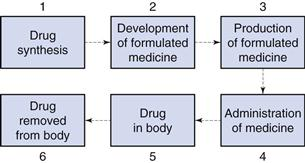Particle size influences the production of many formulated medicines (stage 3, Fig. 9.1) as discussed in later chapters of this book. For instance, both tablets and capsules are manufactured using equipment that controls the mass of drug (and other solid excipients) by volumetric filling. Therefore, any interference with the uniformity of fill volumes may alter the mass of drug incorporated into the tablet or capsule and hence adversely affect the content uniformity of the medicine. Powders with different particle sizes have different flow and packing properties, which alter the volumes of powder during each encapsulation or tablet compression event. In order to avoid such problems, the particle sizes of drugs and other powders may be defined during formulation so that problems during production are avoided.

Following administration of the medicine (stage 4, Fig. 9.1), the dosage form should release the drug into solution at the optimum rate. This depends on several factors, one of which will be the particle size of drug, which is inversely related to particle size as described by the Noyes and Whitney equation, outlined in detail in Chapter 2. Thus, reducing the size of particles will generally increase the rate of dissolution, which can have a direct impact on bioavailability and subsequent drug handling by the body (stages 5 and 6). For example, the drug griseofulvin has a low solubility by oral administration, but is rapidly distributed following absorption; reducing the particle size increases the rate of dissolution and consequently the amount of drug absorbed. However, a reduction in particle size to improve dissolution rate and hence bioavailability is not always beneficial. For example, reducing the particle size of nitrofurantoin increases its dissolution rate, and may consequently produce adverse side-effects because of its more rapid absorption. The effect of particle size on bioavailability is discussed more fully in Chapter 20.

It is clear from the lifetime of a drug outlined above that the knowledge and control of particle size are of importance for both the production of medicines containing particulate solids and the efficacy of such medicines following administration.

The pharmaceutical scientist may not necessarily need to know the precise size of particles intended for a particular purpose, rather a size range may be specified, and consequently powders are frequently graded based on the size of the particles of which they are comprised. For instance:

Pharmacopoeial definitions of grades of powders, and the methods employed to separate particles, by size, are discussed in detail in Chapter 10.

Whilst this chapter refers to ‘particle size’ and ‘particle size analysis’ and the above discussion relates to solid particles, it should be noted that many of the concepts discussed in this chapter apply equally to pharmaceutical systems where it is necessary to determine the size of a dispersed liquid, rather than solid phase, for instance in emulsions and aerosol sprays.

## Particle size

### Dimensions

Describing the size of irregularly shaped particles, as usually encountered in pharmaceutical systems, is a challenge. To adequately describe such a particle would require measurement of no fewer than three dimensions. Frequently, though, it is advantageous to have a single number to describe the size of particles. For instance, when milling powders for inclusion in a pharmaceutical formulation, production and quality assurance staff will want to know if the mean size is approximately the same, larger or smaller than for previous milling procedures of the same material. To overcome the problem of describing a three-dimensional particle with a single number, we employ the concept of the equivalent sphere. Using this approach, a particle is considered to approximate to a sphere: some property of the particle is measured and related to a sphere, the diameter of which can be quoted. Because the measurement is then based on a hypothetical sphere, which represents only an approximation to the true size and shape of the particle, the dimension is referred to as the equivalent sphere diameter or equivalent diameter of the particle.

### Equivalent sphere diameters

It is possible to generate more than one sphere which is equivalent to a given irregular particle shape. Figure 9.2 shows the two-dimensional projection of a particle with two different diameters constructed about it.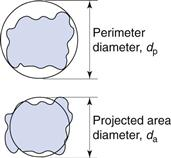The projected area diameter is based on a circle of equivalent area to that of the projected image of a particle; the perimeter diameter is based on a circle having the same perimeter as the particle. Unless the particles are unsymmetrical in three dimensions then these two diameters will be independent of particle orientation.

This is not true for Feret’s and Martin’s diameters (Fig. 9.3), the values of which are dependent on both the orientation and the shape of the particles. These are statistical diameters which are averaged over many different orientations to produce a mean value for each particle diameter. Feret’s diameter is determined from the mean distance between two parallel tangents to the projected outline of the particle. Martin’s diameter is the mean chord length of the projected particle perimeter, which can be considered as the boundary separating equal particle areas (A and B in Fig. 9.3).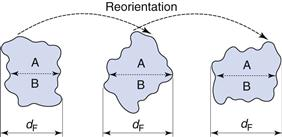It is also possible to determine the equivalent sphere diameters of particles based on other factors such as volume, surface, sieve aperture, sedimentation characteristics, etc. Some of the more commonly used equivalent sphere diameters are defined in Table 9.1; there are others. In general, the method used to determine particle size dictates the type of equivalent diameter that is measured. This is explained for each particle size analysis method described later in this chapter. Inter-conversion of the various equivalent particle sizes may be carried out and this can be done mathematically or automatically as part of the size analysis.

Table 9.1

Equivalent diameters of irregular particles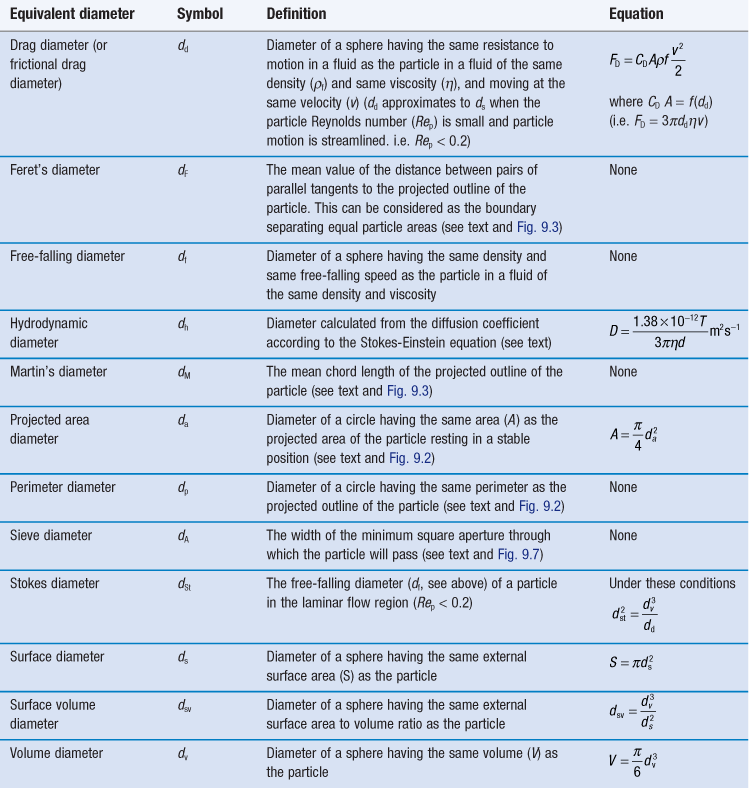### Particle size distribution

A particle population which consists of spheres or equivalent spheres of the same diameter is said to be monodispersed or monosized, and its characteristics can be described by a single diameter or equivalent sphere diameter.

However, it is unusual for particles to be completely monodispersed and such a sample will rarely, if ever, be encountered in a pharmaceutical system. Most powders contain particles with a range of different equivalent diameters, i.e. they are polydispersed or heterodispersed. In order to be able to define a size distribution or compare the characteristics of two or more powders consisting of particles with many different diameters, the size distribution can be broken down into different size ranges, which can be presented in the form of a histogram plotted from data such as those in Table 9.2.

Table 9.2

Frequency and cumulative frequency distribution data for a nominal particle size analysis procedure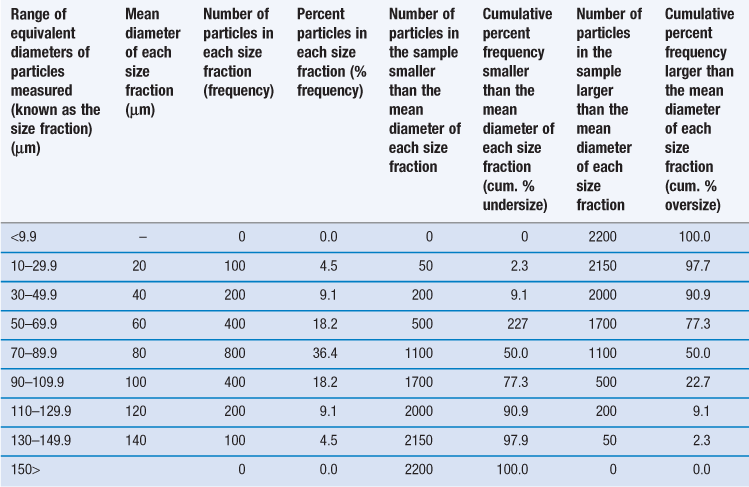Such a histogram presents an interpretation of the particle size distribution and enables the percentage of particles having a given equivalent diameter to be determined. A histogram allows different particle size distributions to be compared. For example, the histogram in Figure 9.4a is a representation of particles that are normally distributed symmetrically about a central value. The peak frequency value, known as the mode, separates the normal curve into two identical halves, because the size distribution is fully symmetrical, i.e. the data in Table 9.2 are normally distributed.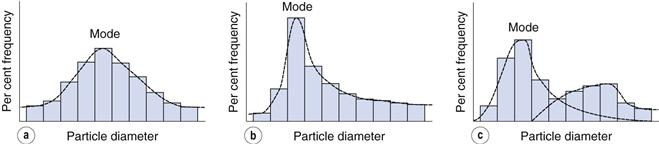Not all particle populations are characterized by symmetrical, or normal, size distributions and the frequency distributions of such populations are said to be skewed. The size distribution shown in Figure 9.4b contains a large proportion of fine particles. A frequency curve such as this, with an elongated tail towards higher size ranges is said to be positively skewed; the reverse case exhibits negative skewness. These skewed distributions can sometimes be normalized by replotting the equivalent particle diameters using a logarithmic scale, and are thus usually referred to as log-normal distributions.

In some size distributions more than one mode occurs: Figure 9.4c shows a bimodal frequency distribution for a powder which has been subjected to milling. Some of the coarser particles from the unmilled population remain unbroken and produce a mode towards the highest particle size, whereas the fractured (size reduced) particles have a new mode which appears lower down the size range.

An alternative to the histogram representation of particle size distribution is obtained by sequentially adding the percent frequency values, as shown in Table 9.2, to produce a cumulative percent frequency distribution. If the addition sequence begins with the coarsest particles, the values obtained will be cumulative percent frequency undersize (or more commonly cumulative percent undersize); the reverse case produces a cumulative percent oversize.

It is possible to compare two or more particle populations using the cumulative distribution representation. Figure 9.5 shows two cumulative percent frequency distributions. For example, the size distribution in Figure 9.5a shows that this powder has a larger range or spread of diameters than the powder represented in Figure 9.5b. The median particle diameter corresponds to the point that separates the cumulative frequency curve into two equal halves, above and below which 50% of the particles lie (point a in Fig. 9.5). Just as the median divides a symmetrical cumulative size distribution curve into two equal halves, so the lower and upper quartile points at 25% and 75% divide the upper and lower ranges of a symmetrical curve into equal parts (points b and c, respectively, in Fig. 9.5).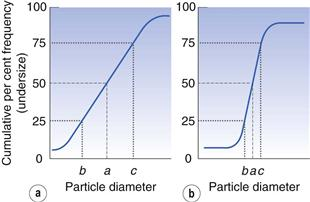#### Statistical methods to summarize size distribution data

Although it is possible to describe particle size distributions qualitatively, it is always more satisfactory to compare particle size data quantitatively. This is made possible by summarizing the distributions using statistical methods.

In order to quantify the degree of skewness of a particle population, the interquartile coefficient of skewness (IQCS) can be determined as follows:(9.1)

where a is the median diameter and b and c are the lower and upper quartile points (see Fig. 9.5).

The IQCS can take any value between −1 and +1. If the IQCS is zero then the size distribution is practically symmetrical between the quartile points. To avoid ambiguity in interpreting values for IQCS, a large number of size intervals is required.

To quantify the degree of symmetry of a particle size distribution, a property known as kurtosis can be determined. The symmetry of a distribution is based on a comparison of the height or thickness of the tails and the ‘sharpness’ of the peaks with those of a normal distribution. ‘Thick’-tailed, ‘sharp’ peaked curves are described as leptokurtic, whereas ‘thin’-tailed, ‘blunt’ peaked curves are platykurtic and the normal distribution is mesokurtic.

The coefficient of kurtosis, k (Eqn 9.2), has a value of 0 for a normal curve, a negative value for curves showing platykurtosis and positive values for leptokurtic size distributions: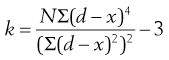(9.2)

where d is any particle diameter, x is mean particle diameter and N is number of particles. Again, a large number of data points is required to provide an accurate analysis.

### Mean particle sizes

As established above, it is impossible for any single number to fully describe the size distribution of particles in a real pharmaceutical system. However, for the sake of simplicity, pharmaceutical scientists wish to employ a single number to represent the mean size of a powder sample. The mean of the particle population, the median (Fig. 9.5) and the mode (Fig. 9.4) are all measures of central tendency. They provide a single value near the middle of the size distribution that attempts to represent a central particle diameter. Whereas the mode and median diameters can be obtained for an incomplete particle size distribution, the mean diameter can only be determined when the size distribution is complete and the upper and lower size limits are known. It is possible to define the ‘mean’ particle size in several ways.

#### Arithmetic means

Arithmetic means are obtained by summating a particular parameter for all the individual particles in a sample and dividing the value achieved by the total number of particles. Means can be related to the diameter, surface area, volume or mass of a particle. In the equations below, x is the mean particle size and the subscripts L, S, V and M indicate mean size based on length (diameter), surface area, volume and mass, respectively. ΣdL